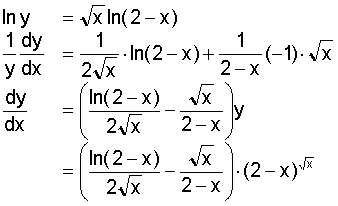# Logarithmic Differentiation

The original problem is y = (2-x)^(sqrt x). If anyone who is rather confident with this could double check this example it would really help me out. Thanks. I attached the link to reduce my typing confusion.

http://archives.math.utk.edu/visual.calculus/3/logdiff.1/a1.gif

SammyS
Staff Emeritus
Homework Helper
Gold Member

The original problem is y = (2-x)^(sqrt x). If anyone who is rather confident with this could double check this example it would really help me out. Thanks. I attached the link to reduce my typing confusion.

http://archives.math.utk.edu/visual.calculus/3/logdiff.1/a1.gif
Here's the image:What is it that looks off to you?

#### Attachments

I can not pinpoint anything. I am aware of the steps of the log diff for other examples on this weeks homework. Prof through it out to the class that this looks off. I cant see it. Should I look deeper?

Looks perfectly fine to me.

Provided you understand the product rule, the chain rule, and the fact that ln(a^b) = b.ln(a), there isn't really anything mysterious here at all.

ok thanks. Perhaps it was thrown out to check our grasp of your aforementioned rules. Thanks for the time.

I threw this out last night, but it is still getting some pushback.

How does this look? Right or a little off? I placed the problem and solution link since I can not figure out how to import the image.

http://archives.math.utk.edu/visual....gdiff.1/a1.gif [Broken]

Last edited by a moderator:
This is what I get when trying to look at the image:

The original problem is...

y = (2-x)^sqrt(x)

And you want the derivative?

yes, using logarithmic differentiation solve the problem.

In general, when you have something like $$f(x)^{g(x)}$$ you can find the derivative like this, which should give you what you need to solve your specific problem where log is the natural logarithm:

$$y=f(x)^{g(x)}$$
$$\log{y}=g(x)\log{f(x)}$$
Implicitly differentiate both sides:
$$\frac{dy}{y}=g'(x)\log{f(x)}dx+\frac{g(x)f'(x)}{f(x)}dx$$
$$y'=y\left(g'(x)\log{f(x)}+\frac{g(x)f'(x)}{f(x)} \right)$$
$$y'=f(x)^{g(x)}\left(g'(x)\log{f(x)}+\frac{g(x)f'(x)}{f(x)}\right)$$

yes, using logarithmic differentiation solve the problem.

It looks fine.

DonAntonio

Your efforts to spread this question to a larger audience have obviously been quashed, as it looks like it's been merged into your original thread!
I threw this out last night, but it is still getting some pushback.
What exactly do you mean by "some pushback"? Has your Prof said something? If so, what? Is he/she worried about the domain/range not being real etc?

Even the God Almighty that is Woolfy-Alfa agrees that this is the correct solution.

Last edited: